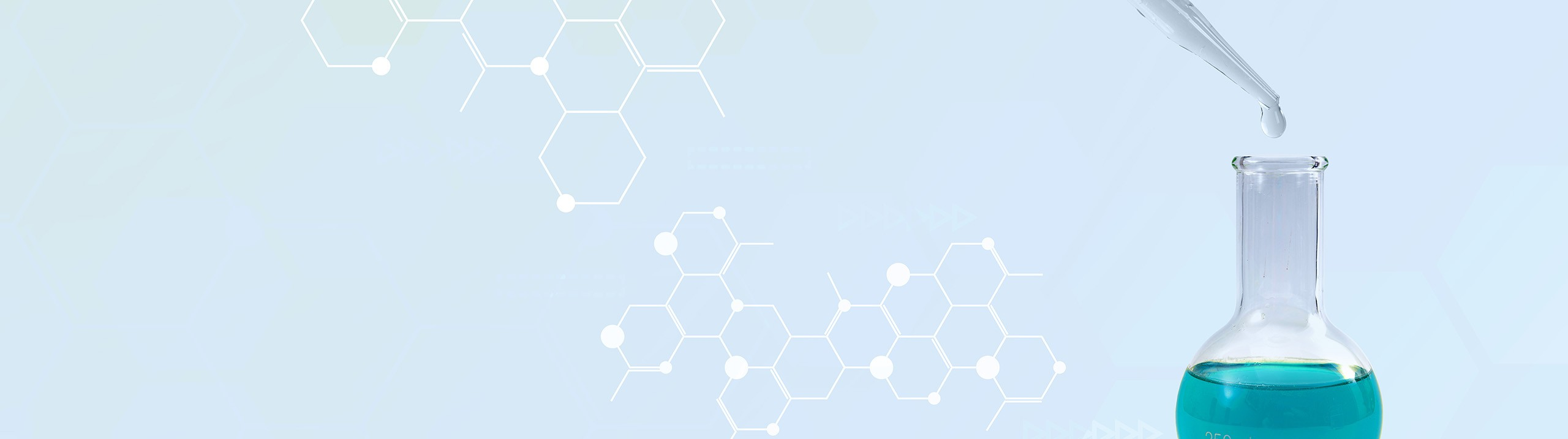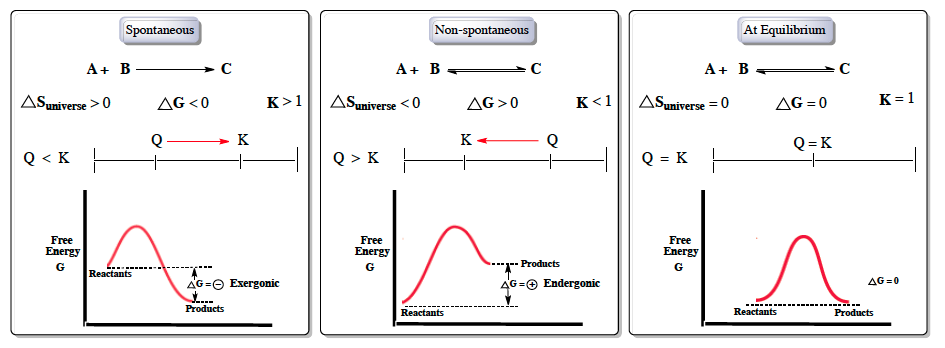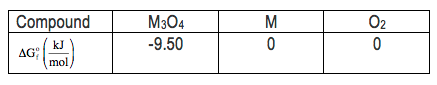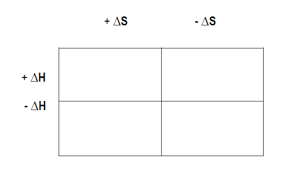Start typing, then use the up and down arrows to select an option from the list.# Analytical Chemistry

Learn the toughest concepts covered in your Analytical Chemistry class with step-by-step video tutorials and practice problems.

Chemical Equilibrium

# Chemical Thermodynamics: Gibbs Free Energy

Gibbs Free Energy represents the maximum amount of work that can be done by a thermodynamic reaction at constant pressure and temperature.

## Understanding Gibbs Free Energy

1

#### concept

Gibbs Free Energy6m
Play a video:2

#### example

Gibbs Free Energy3m
Play a video:
So, recall that a spontaneous reaction is one that happens naturally without the need of outside energy, whereas a non spontaneous reaction is one that doesn't normally occur unless some outside forces in play. So, if we take a look here, we have to figure out which of the following is an example of a non spontaneous process. Alright, so first we have ice melting at room temperature. Now we know that as long as the temperatures above zero degrees Celsius, which room temperature is, ice will naturally melt because this happens in real world scenarios, it is a spontaneous process. Next sodium metal reacting violently with water. Uh this one probably not as well known, just remember here that when it comes to group one A and two, a metals, because of the low number of valence electrons they have, they will violently react with water in order to lose an electron to become more like a noble gas. So here this is true, because it happens naturally in the real world. It's a spontaneous process here rusting of iron at room temperature. So, old cars when it rains, they can rust. This is a natural process that happens every day. And because of that, it's a spontaneous process, a ball rolling downhill. So a ball can naturally roll down a hill using its momentum as well as gravity to push itself down that hill, we can see that this happens um just by imagining it, you can take a ball and throw it down a hill, you'll see it roll down naturally. So it's a spontaneous process. Now, even if you weren't able to determine if the initial four were spontaneous or not, you should know that E is a non spontaneous process here. It says water freezing at room temperature, Room temperature is much too warm for water to freeze. Water has to be at 0°C or lower in order to begin to freeze. So we should know that this makes no sense. It's not a natural occurrence. It could only happen if we invested some outside energy. For example, we have a refrigerator where we put a nice tray in the refrigerator is in a room at room temperature, but inside the refrigerator it's much lower than that. They're therefore water could freeze. So the last option here, we're going to say, does not naturally occur. Water will not freeze at room temperature under natural conditions. Therefore, it is the process that is non spontaneous. Also remember, as we set up above, spontaneous reactions move in the four directions, they never move in the in the reverse direction, because if it's spontaneous, it's gonna move forward. It is non reversible or irreversible. Non spontaneous processes are not spontaneous in the four direction, so they tend to move in the reverse direction to become spontaneous. So non spontaneous processes are reversible

## Gibbs Free Energy Calculations

3

#### example

Gibbs Free Energy Calculations 16m
Play a video:4

#### example

Gibbs Free Energy Calculations 13m
Play a video:
So here we're told for the reaction of two moles of carbon graphite reacting with one mole of hydrogen gas produces one mole of ethylene gas. Here we're told that gives free energy under standard conditions is 209.2 killer jewels at 25 degrees Celsius. Now we're also told that if the partial pressure of hydrogen gas equals 100 atmospheres and the partial pressure of ethylene gas equals 0.10 atmospheres, calculate gibbs free energy under nonstandard conditions for the reaction. So here we're dealing with gibbs free energy under standard conditions and nonstandard conditions. So the equation that connects them together is gibbs, free energy under nonstandard conditions equals gives free energy under standard conditions plus R T L N Q. Remember Q is just your reaction quotient, it's equal to products overreacting, just like your equilibrium constant and just like your equilibrium constant, we're gonna ignore solids and liquids. So here this would be C two H two Divided by H two. Here we're gonna plug in their partial pressures, technically again we're dealing with pressure. So technically we should only reserve brackets when we're dealing with concentrations. So technically it's P C two, H two. And then ph two here on the bottom. So here my Q would be 0.10 divided by 100 for Q. Now here are has jewels in its units. So we should convert the 209.2 killer jewels into jewels. So remember, one killer jewel is equal to 1000 jewels. So that's 209,200 jewels. So take that number plug it in. Plus our which is 8.314 jules over moles. Times K Temperature has to be in Kelvin. So to the 25°C, you add to 73.15 Which comes out to 298.15 Kelvin And then Ln of 0.10 divided by 100. So here When you work this out, that's gonna come out to be 19, jules as our units. But all the answers here are in killed jules. So we do one more conversion. So remember one killer jewel is equal to 1000 jewels. So that's 192.077 killer jewels which rounds up to 1 92.1 killer jewels. Making option A are correct choice. So just remember in this question, we're dealing with gibbs, free energy, both under standard conditions and nonstandard conditions. So you just have to recall what equation connects those two variables together and then plugging desired units to find your answer at the end
5

#### example

Gibbs Free Energy Calculations 28m
Play a video: